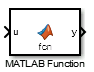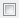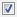# MATLAB Function

MATLAB 代码包含在生成可嵌入式 C 代码的模型中

•HDL Coder / User-Defined Functions

## 描述

```function [mean,stdev] = stats(vals) % Calculates a statistical mean and a standard % deviation for the values in vals. len = length(vals); mean = avg(vals,len); stdev = sqrt(sum(((vals-avg(vals,len)).^2))/len); plot(vals,"-+"); function mean = avg(array,size) mean = sum(array)/size;```MATLAB Function 模块基于分析来生成有效的可嵌入式代码，该分析可确定每个变量的大小、类和复/实性。该分析施加了以下限制：

• 首次对变量赋值时会定义其大小、类和复/实性。

• 在初始赋值后不能为变量属性重新赋值，但在代码中可以针对不同用途使用可变大小的数据或重复使用变量。

请参阅变量属性的重新赋值

• `plus``minus``power` 等算术运算符。有关详细信息，请参阅数组与矩阵运算

MATLAB Function 模块中不允许递归调用。

## 端口

### 输入

MATLAB 支持但 Simulink 不支持的数据类型可能无法在 Simulink 模型和 MATLAB Function 模块内的函数之间传递。这些类型可在 MATLAB Function 模块内使用。

## 参数

### 常设

• - 不显示端口标签。

• FromPortIcon - 显示输入和输出变量的名称。

• FromPortBlockName - 显示输入和输出变量的名称。

• SignalName - 如果连接到端口的信号已命名，则显示信号名称。否则，显示变量的名称。

#### 编程用法

 参数：`ShowPortLabels` 类型：字符串标量或字符向量 值：`"none"` | `"FromPortIcon"` | `"FromPortBlockName"` | `"SignalName"` 默认值：`"FromPortIcon"`

• ReadWrite - 允许打开和修改 MATLAB Function 模块内容。

• ReadOnly - 允许打开 MATLAB Function 模块。

• NoReadOrWrite - 禁止打开或修改 MATLAB Function 模块。

#### 编程用法

 参数：`Permissions` 类型：字符串标量或字符向量 值：`"ReadWrite"` | `"ReadOnly"` | `"NoReadOrWrite"` 默认值：`"ReadWrite"`

•off - 不尝试消除任何包含原子单元的人为代数环。

•on - 尝试消除任何包含原子单元的人为代数环。

#### 编程用法

 参数：`MinAlgLoopOccurrences` 类型：字符串标量或字符向量 值：`"off"` | `"on"` 默认值：`"off"`

• 如果 MATLAB Function 模块中的变量能以不同速率运行，请将采样时间指定为继承 (`-1`)。

• 如果变量必须以相同的速率运行，请指定与此速率对应的采样时间 `Ts`

#### 编程用法

 参数：`SystemSampleTime` 类型：字符串标量或字符向量 值：`"-1"` | `"[Ts 0]"` 默认值：`"-1"`

### 代码生成

Simulink Coder 将基于模型中存在的 MATLAB Function 模块的类型和实例数为您的系统选择最佳格式。

Simulink Coder 无条件地内联 MATLAB Function 模块。

Simulink Coder 在单独的文件中显式生成单独的函数。具有此设置的 MATLAB Function 模块生成的函数可能具有取决于代码生成中的函数接口的参数。您可以分别使用代码生成中的参数函数名称文件名(无扩展名) 来命名生成的函数和文件。这些函数不可重入。

Simulink Coder 可以生成带参数的函数，当模型中包含模块的多个 MATLAB Function 实例时，将允许重用 MATLAB Function 模块的代码。

#### 提示

• 当您要将一个 MATLAB Function 模块的多个实例表示为一个可重用函数时，您可以将每个实例指定为自动可重用函数。最好是使用其中一个，因为使用两个将生成两个可重用函数，每个指定一个函数。仅当可以重用时，这些选项的结果才会有区别。选择自动则不允许控制 MATLAB Function 模块代码的函数或文件名。

• 可重用函数自动选项都会确定是否存在 MATLAB Function 模块的多个实例，以及是否可以重用代码。当无法重用代码时，这些选项的行为会有所不同。在这种情况下，自动生成内联代码，或者在禁止内联的情况下为每个模块实例生成一个单独的函数。

• 如果您在生成的代码处于源代码管理状态下时选择了可重用函数，请将文件名选项设置为使用子系统名称使用函数名称用户指定。否则，点您修改模型时，代码文件的名称就会改变，从而无法对文件进行源代码管理。

#### 依存关系

• 将此参数设置为不可重用函数可重用函数将启用下列参数：

• 函数名称选项

• 文件名选项

• 用于初始化/终止函数的内存段（需要 Embedded Coder® 和基于 ERT 的系统目标文件）

• 用于执行函数的内存段（需要 Embedded Coder 和基于 ERT 的系统目标文件）

• 将此参数设置为不可重用函数将启用具有独立数据的函数（需要 Embedded Coder 许可证和基于 ERT 的系统目标文件）。

#### 编程用法

 参数：`RTWSystemCode` 类型：字符串标量或字符向量 值：`"Auto"` | `"Inline"` | `"Nonreusable function"` | `"Reusable function"` 默认值：`"Auto"`

## 模块特性

 数据类型 `Booleana` | `busa` | `doublea` | `enumerateda` | `fixed pointa` | `halfa` | `integera` | `singlea` | `stringa` 直接馈通 `是a` 多维信号 `是a` 可变大小信号 `是a` 过零检测 `否` a 实际支持的数据类型或功能取决于模块实施。